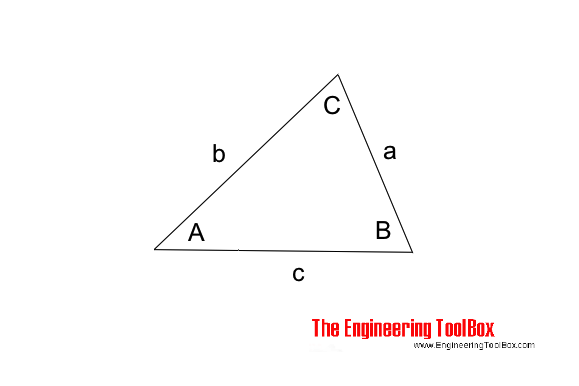Engineering ToolBox - Resources, Tools and Basic Information for Engineering and Design of Technical Applications!

# Law of Tangents

## Triangles and law of tangents.

The "Law of Tangents" can be used to calculate the angles or sides of a triangle.### Law of Tangents

The "Law of Tangents" can be expressed as

(a + b) / (a - b) = tan 1/2 (A + B) / tan 1/2 (A - B)                         (1)

where

a, b and c = length of sides in triangle (m, ft ...)

A, B and C = angles in the triangle (degrees)

### Pythagorean theorem

The Pythagorean theorem can be expressed as

a2 + b2 = c2                 (2)

### Law of sines

The Law of sines can be expressed as

a / sin(A) = b / sin(B) = c / sin(C)                (3)

### Angles

If the length of all three sides in the triangle are known - the angles can be calculated as

A = arccos((b2 + c2 - a2) / (2 b c))                     (4a)

B = arccos((a2 + c2 - b2) / (2 a c))                     (4b)

C = arccos((a2 + b2 - c2) / (2 a b))                     (4c)

## Related Topics

• ### Mathematics

Mathematical rules and laws - numbers, areas, volumes, exponents, trigonometric functions and more.

## Related Documents

• ### Angle Calculator - Carpenter's Square

Calculate angles with a straight board across carpenter's square.
• ### Law of Cosines

One side of a triangle when the opposite angle and two sides are known.
• ### Law of Sines

Calculate the angles in a generic triangle.
• ### Miter Saw - Calculate Sawing Angle

Calculate miter saw protractor angles for skirting and decorative mouldings work.
• ### Oblique Triangle

Calculate oblique triangles.
• ### Pythagorean Theorem

Verifying square corners.
• ### Right Angled Triangle

Right angled triangle equations.
• ### Squaring with Diagonal Measurements

A rectangle is square if the lengths of both diagonals are equal.
• ### Triangle

Triangle analytical geometry.
• ### Trigonometric Functions

Sine, cosine and tangent - the natural trigonometric functions.

## Engineering ToolBox - SketchUp Extension - Online 3D modeling!

Add standard and customized parametric components - like flange beams, lumbers, piping, stairs and more - to your Sketchup model with the Engineering ToolBox - SketchUp Extension - enabled for use with older versions of the amazing SketchUp Make and the newer "up to date" SketchUp Pro . Add the Engineering ToolBox extension to your SketchUp Make/Pro from the Extension Warehouse !

We don't collect information from our users. More about

## Citation

• The Engineering ToolBox (2020). Law of Tangents. [online] Available at: https://www.engineeringtoolbox.com/law-tangents-d_2178.html [Accessed Day Month Year].

Modify the access date according your visit.

10.2.10